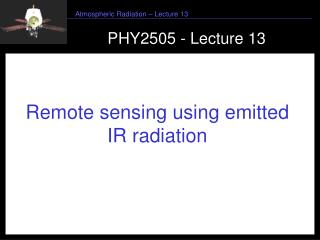DownloadDownload PresentationPHY2505 - Lecture 13

# PHY2505 - Lecture 13

Télécharger la présentation## PHY2505 - Lecture 13

- - - - - - - - - - - - - - - - - - - - - - - - - - - E N D - - - - - - - - - - - - - - - - - - - - - - - - - - -
##### Presentation Transcript

1. PHY2505 - Lecture 13 Remote sensing using emitted IR radiation

2. Liou 7.4.1:Revision Solution: Where Can assume: emissivity = 1, m=1 Why?

3. Liou 7.4.1:Monochromatic transmittance, T, and weighting function Change to pressure co-ordinates: Recall Giving:

4. Liou 7.4.1: Instrument response function • Need to convolve the emitted radiance with a function to represent the instrument response: a “slit” function • instrument has only a limited spectral bandwidth • Assume spectral interval is small so replace with • Then • With

5. Liou 7.4.1: Temperature and gas retrievals Surface temperature Temperature profiles Atmospheric window: 800-1200cm-1 Gas phase profiles CO2, 15um, 4.3um O3 9.6um H2O 6.3um

6. Liou 7.4.2: Surface temperature determination Define mean atmospheric temperature and simplify for the case where you are measuring in the window region: Where i=v, and transmission, is mainly reduced by water vapour and can be approximated by To subtract the atmospheric component (eliminate ) we take two measurements: the “split window” technique

7. Liou 7.4.2: Split window technique Applying the window equation to two channels Want in terms of T2 Expand Planck function in terms of Taylor series with respect to Ta Applying Taylor series expansion and eliminating T-Ta Now replacing T by Tb2 and Ts and using equation for I2 get Radiance expressed as Brightness temperature

8. Liou 7.4.2: Split window technique Now replacing T by Tb2 and Ts and using equation for I2 get With Gives where In practical use, the Planck function is replaced by the Brightness temperature and Ts estimated by Equation for I2

9. Liou 7.4.2: Application to sea surface temperatures From NOAA AVHRR data: Retrieval from regression: In three channels (sum and difference): 10.9, 12.0, 3.7um Satellite measures SKIN temperature (first few mm) In situ buoys are used to provide the coefficients a, b and c, which relate these skin temperatures to bulk temperature of water… (McClain et al, 1985)

10. Liou 7.4.3: Remote sensing of temperature profiles • Kaplan (1959): • Vertical resolution of the temperature field can be inferred from spectral distribution of atmospheric emission. • Wings in a band see deep into the atmosphere while centre sees only the top layer: absorption is strongly peaked in centre, so the mean free path of radiation is less for centre than wings.. • Can select different sounding wavelengths each to be sensitive to different layers..

11. Liou 7.4.3: Illustration of concept Assume (find Ts in practice from preceding analysis) Write Solve for this time taking into account variation across spectral interval using reference wavenumber, vr Express equation for Iv as Fredholm Integral where

12. Liou 7.4.3: Choosing spectral region There are two gases that occur in uniform abundance at altitude up to 100km, which also show emission bands in regions convenient for measurements CARBON DIOXIDE (365ppm) and OXYGEN (volume mixing ratio 0.21)

13. Liou 7.4.3: 15um CO2 band as measured by IRIS NIMBUS Decrease of tropospheric temperature as altitude increases Warming due to stratosphere

14. Liou 7.4.3: Weighting functions • An ideal weighting function would be a delta function: all the contribution from that measurement at a single altitude.. • Weighting functions are constructed from • where T can be calculated using a line-by-line code taking pressure and temperature proilfes into account • CO2 transmittances in the 15um band are due to a number of different lines: the v2 fundamental and hot bands..

15. Liou 7.4.3: Example weighting functions corresponding to 15um band intervals